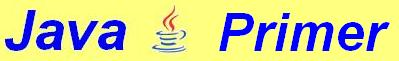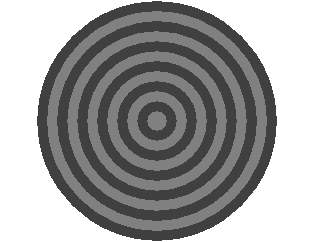4. Conditions, booleans and Dimension

Not many people will want to use their monitor for shooting target. But in this chapter, we are going to make our monitor look like this:In chapter 3 we saw how you can draw concentric circles. But instead of drawOval we are now going to use a method called fillOval, which fills up the circle as well.

true and false: booleans
Going inward, we can alternately draw black and white disks, in other words …

If the disk is black, the next one will be white
If the disk is white, the next one will be black.
It would be nice if we had a variable that can have only two values: black and white.

For this kind of situation Java has the type boolean. A boolean can contain only two values: true and false. Have a look at the following lines, in which we will also learn to know the words if and else
boolean leapYear;
if (numberOfdays == 366)
leapYear = true;
else
leapYear = false;
if (leapYear)
g.drawString ("Leap year", 20, 100);
else
g.drawString ("Ordinary year", 20, 100);
In our shooting-target program, such a condition is also quite handy. In that program we can use a boolean like this:
if (white)
g.setColor (Color.white); // Note the indentation
else
g.setColor (Color.black); // Note the indentation
It is not very hard to understand that g.setColor (Color.white) turns on the colour white. But what is the meaning of if (white)?

Once again, what we see here is a boolean -- a variable which knows only two values: true or false.

Booleans are used in conditions and can be declarered as follows:
boolean correct = true;
boolean lightOn = false;
boolean open = true;
In our program, the colour will be inverted after each painting of a disk. We could do that like this:

if (white == true)
white = false;
else
white = true;

There is, however, a much shorter way:
white = !white;
The exclamation mark (!) is pronounced NOT and means that the content of white is actually inverted. In fact, the three following statements mean exactly the same:
if (!white) . . .
if (white == false) . . .
if (!white == true) . . .

The Dimension class
The dimensions of our applet are normally laid down in the html-file. This could look like this:

<applet code = MyJavaApplet.class
width = 320 height = 240>
</applet>

But sometimes it would be pleasant if we could find out the size of the applet window from within the applet. For this, we can make use of the class Dimension. It is possible to have the dimensions of the applet window put into an object (for instance d) of this Dimension class:

Dimension d = getSize();
This object d has two fields, whose names are width and height. We could put the content of these fields in normal variables, for instance like this:
int width = d.width;     // or other names,
int height = d.height;  // if you like ...
The standard applet window in this tutorial is 320 pixels wide and 240 pixels high. A circle that fits exactly into this window should have a radius of 120 pixels. But if the applet were 400 pixels high and 300 pixels wide, the radius would have to be 150 pixels. We can bring that about in the following way:
if (width > height)
radius = height / 2;
else
radius = width / 2;
Finally, here is the complete program:
// Shooting target that fits exactly into the applet window
import java.applet.*;
import java.awt.*;
public class Target extends Applet {
boolean white = false;
public void paint (Graphics g) {
Dimension d = getSize();
int width = d.width, height = d.height;
int radius = height/2;
if (width < height)
do {
if (white)
g.setColor (Color.white);
else
g.setColor (Color.black);
white = !white;
} while (radius > 0);
}
}

 Exercise 4.1 Write an applet which - depending on the actual dimensions of the applet window - puts one of the following texts on the screen:            The width is greater than the height.            The height is greater than the width.            The width is equal to the height. Exercise 4.2 Write an applet that shows four identical rectangles. The distances on the left, on the right and between the rectangles is equal to the width of each of the rectangles. The distances to the bottom, the top and between them is equal to the height of the rectangles. This ratio must stay correct - whatever the dimensions of the window may be (see example below).

To chapter 5

Main Menu Java Tutorial

To homepage

(c) T.J.H. Luif, 2003